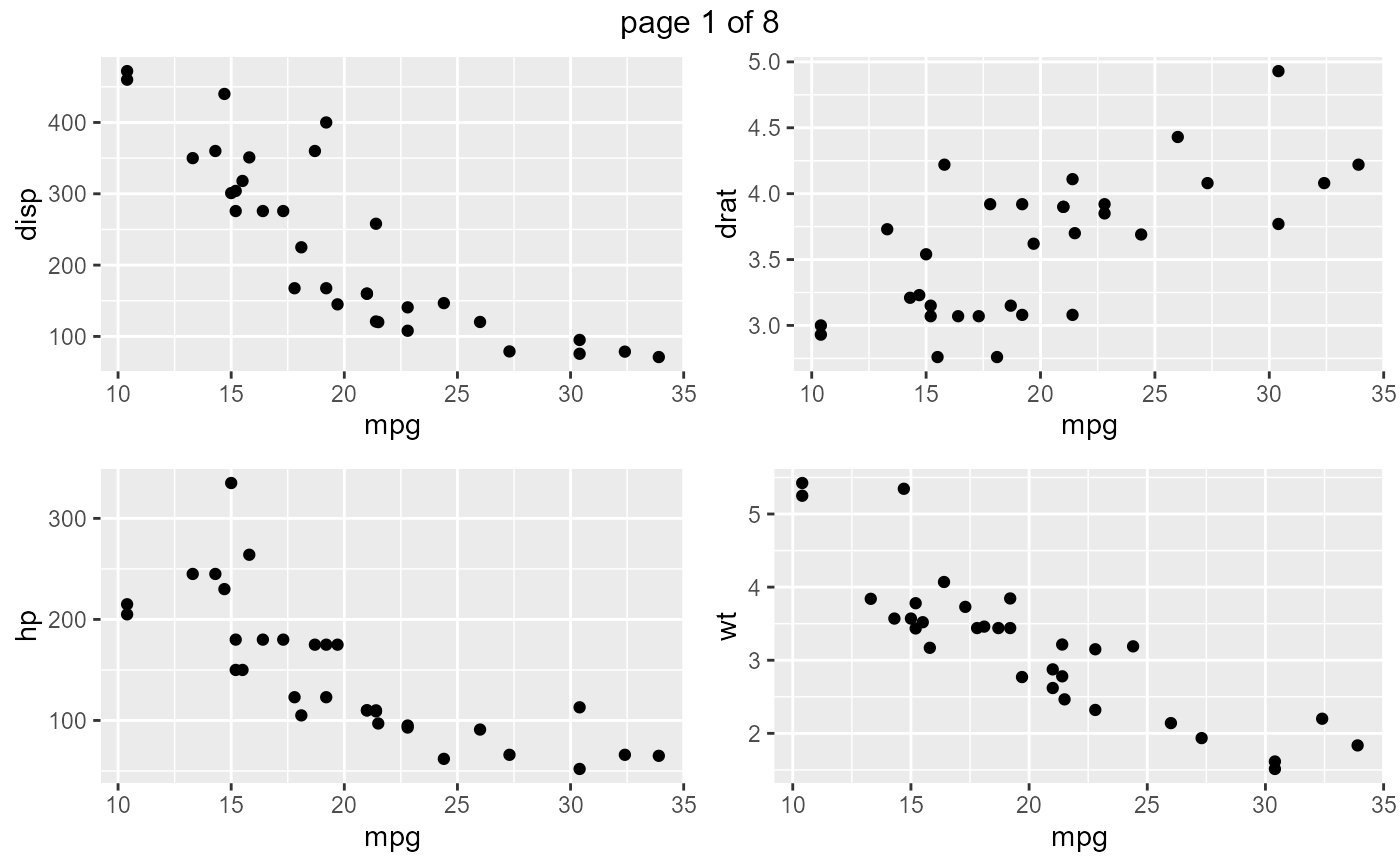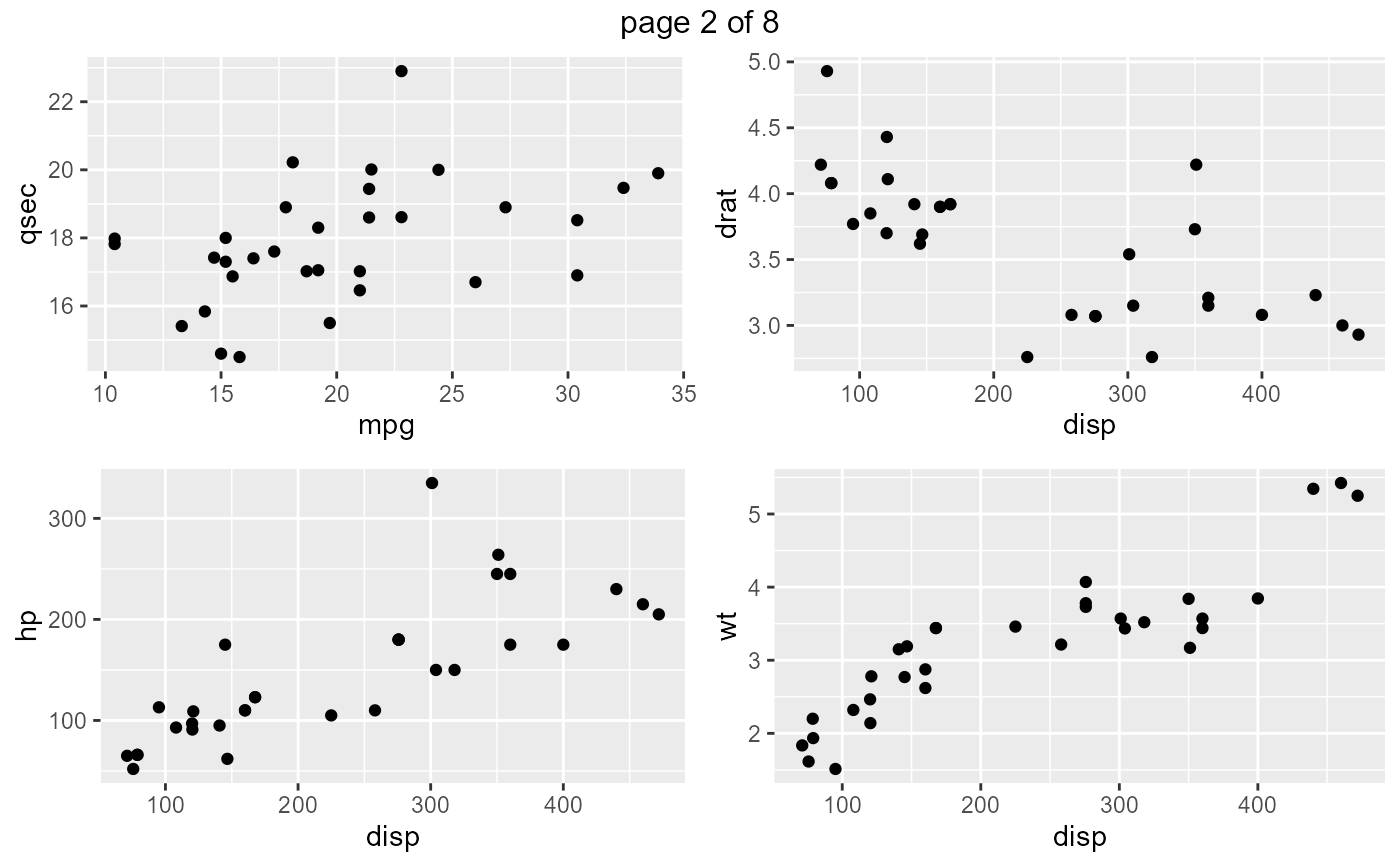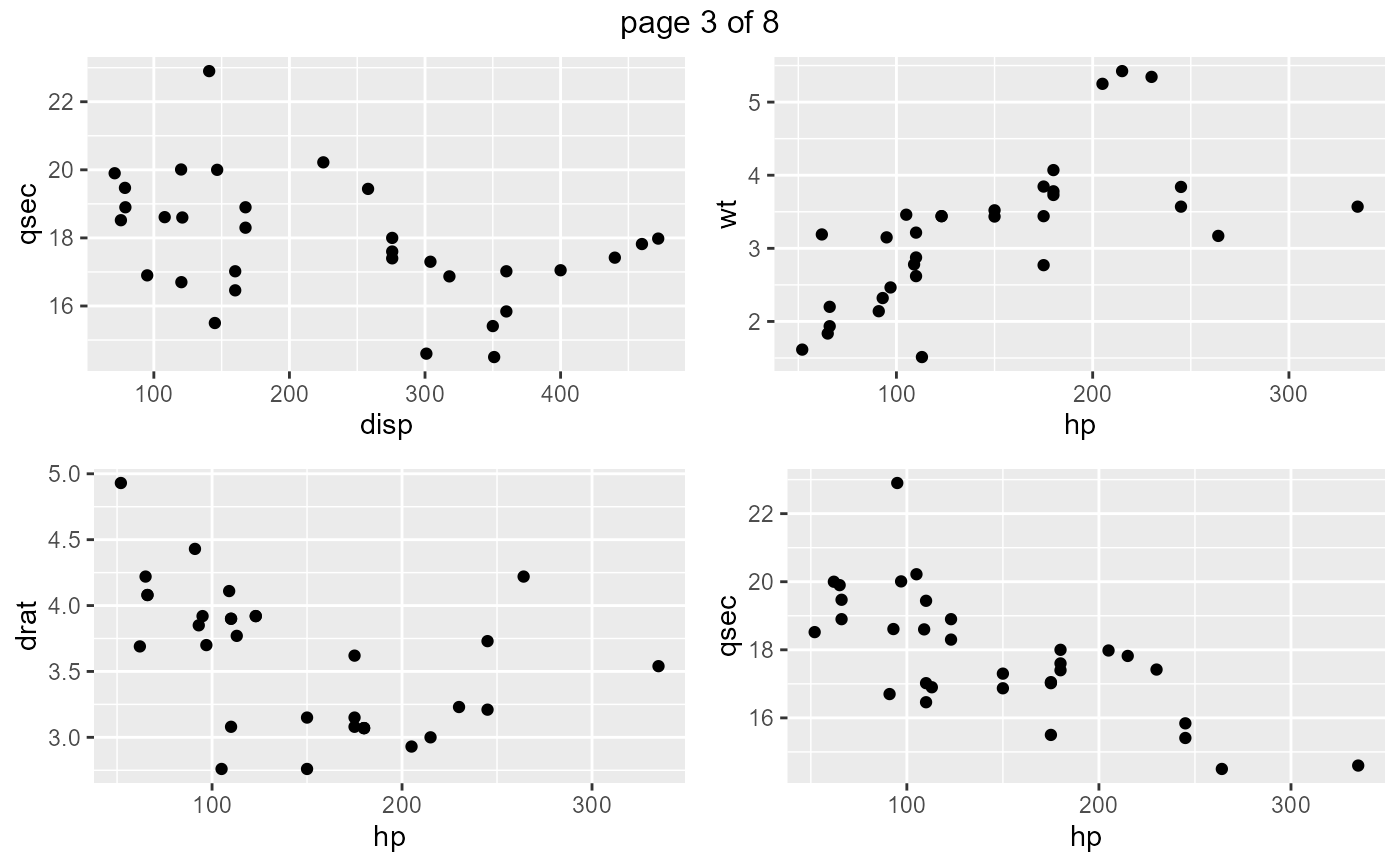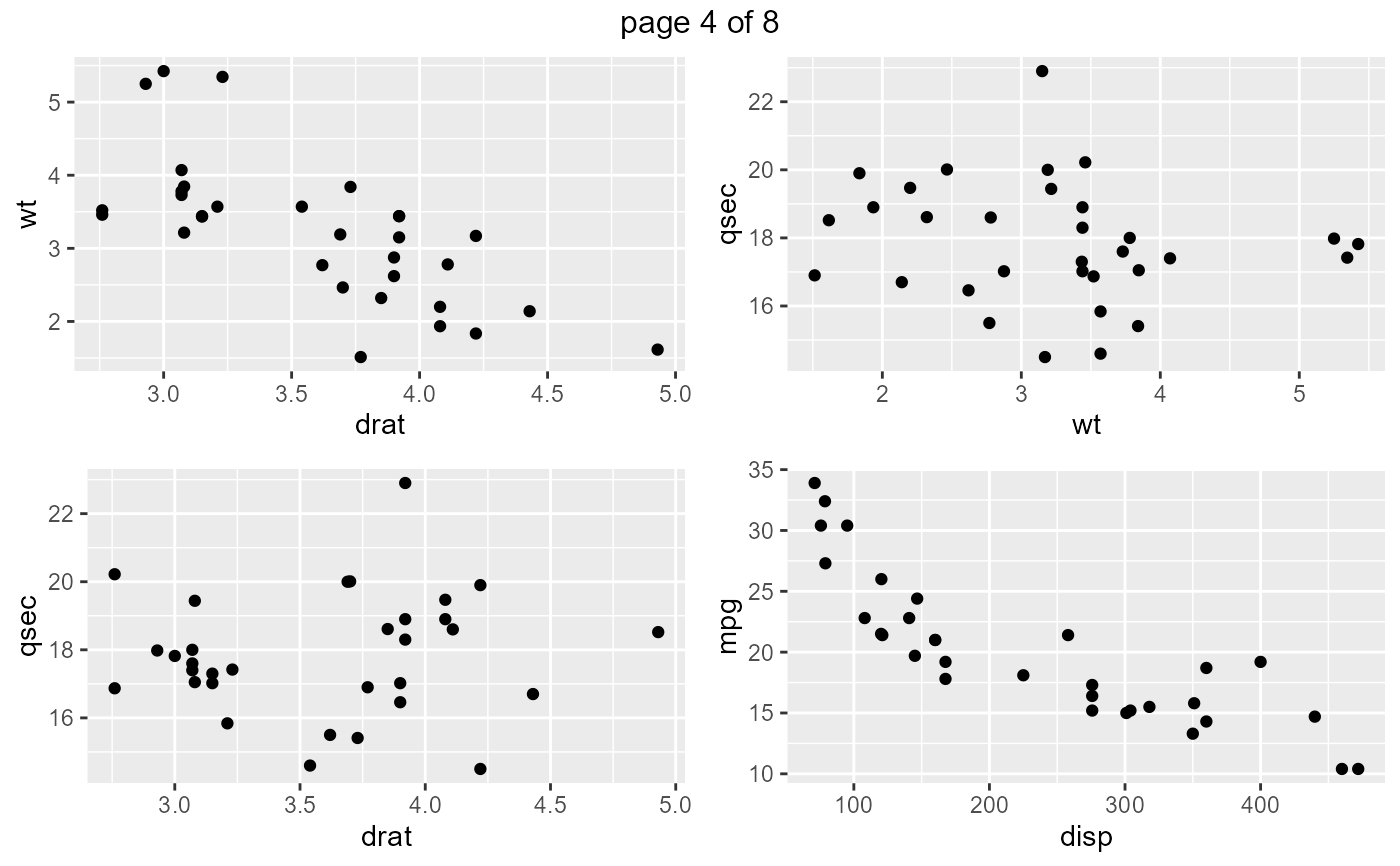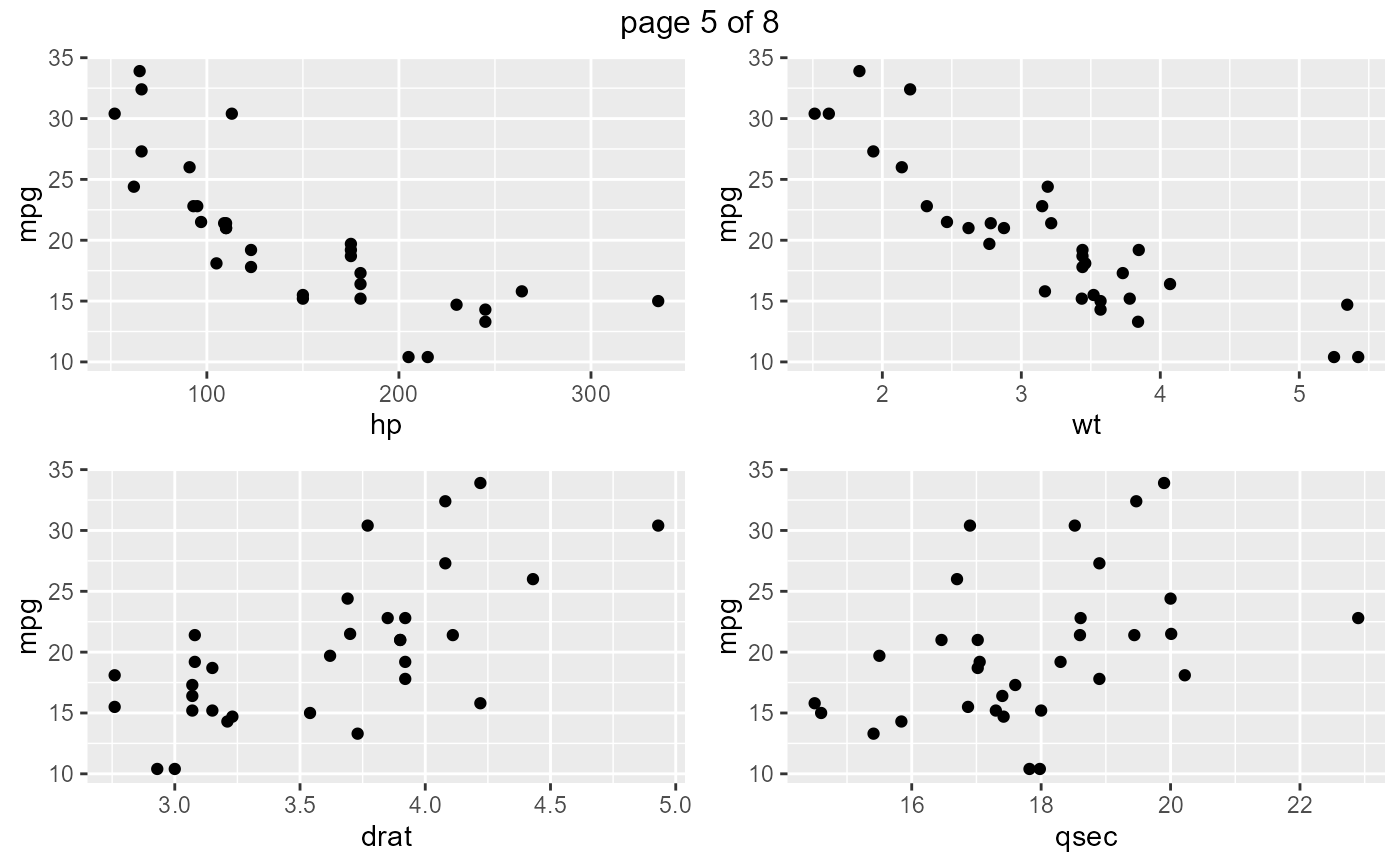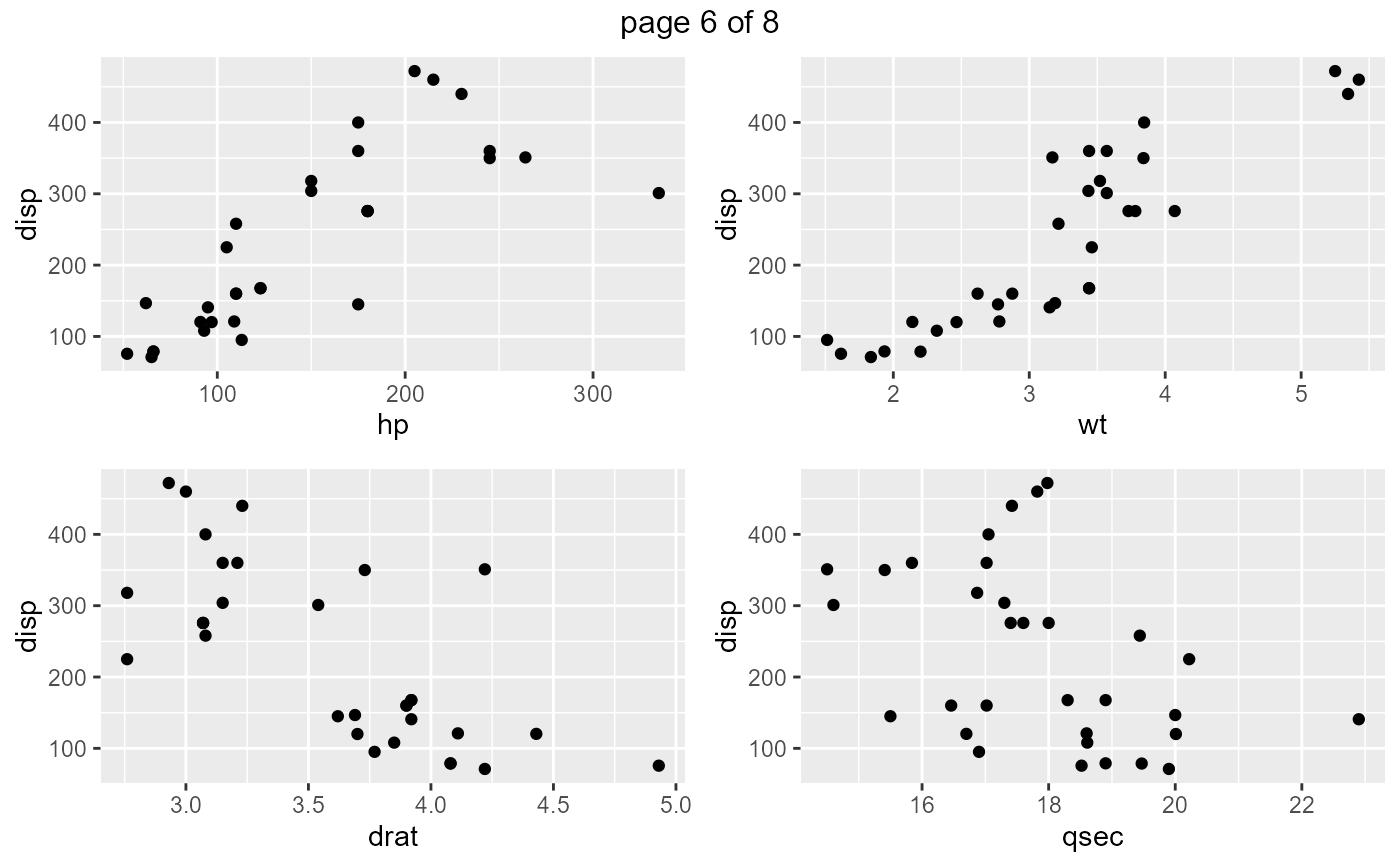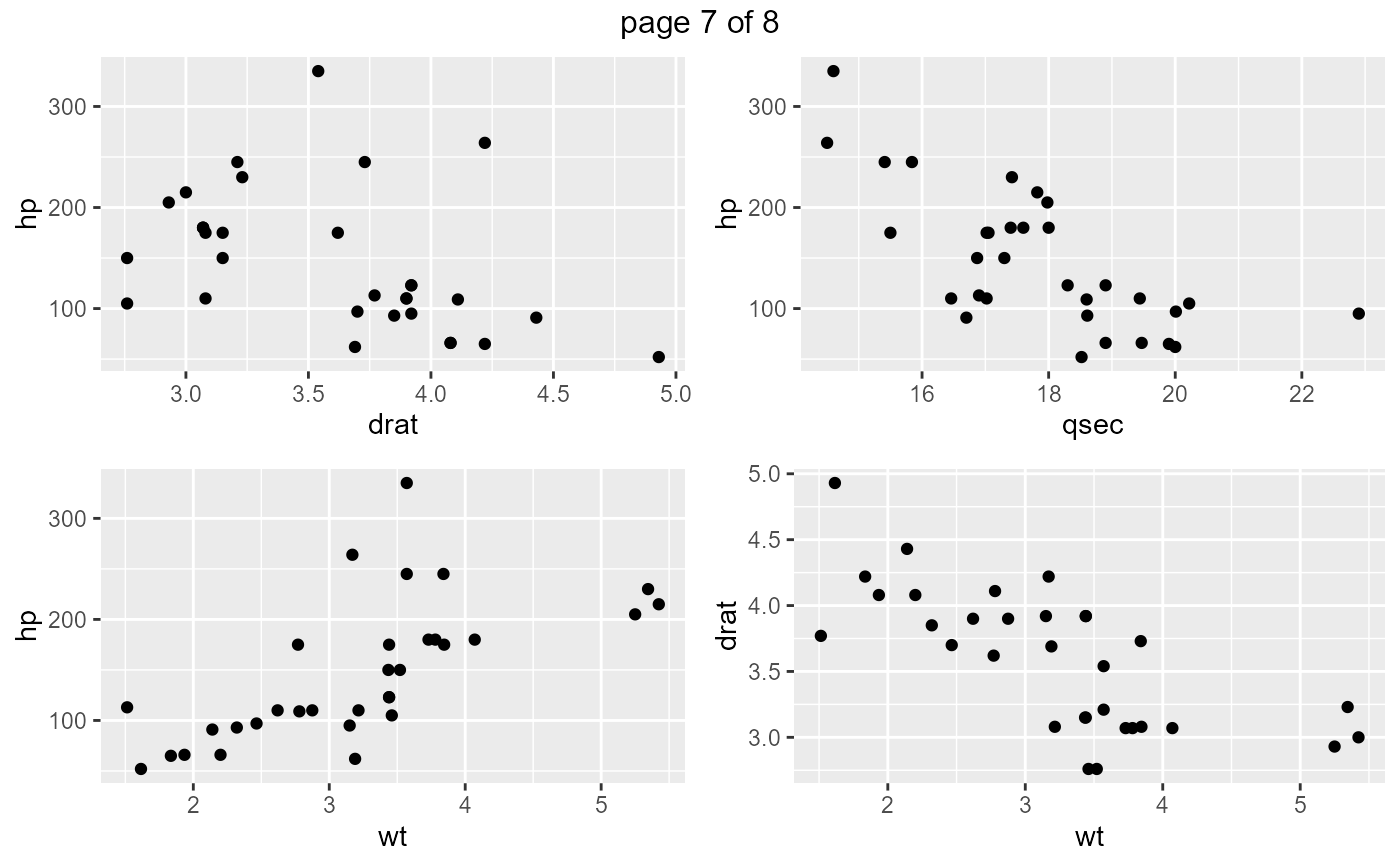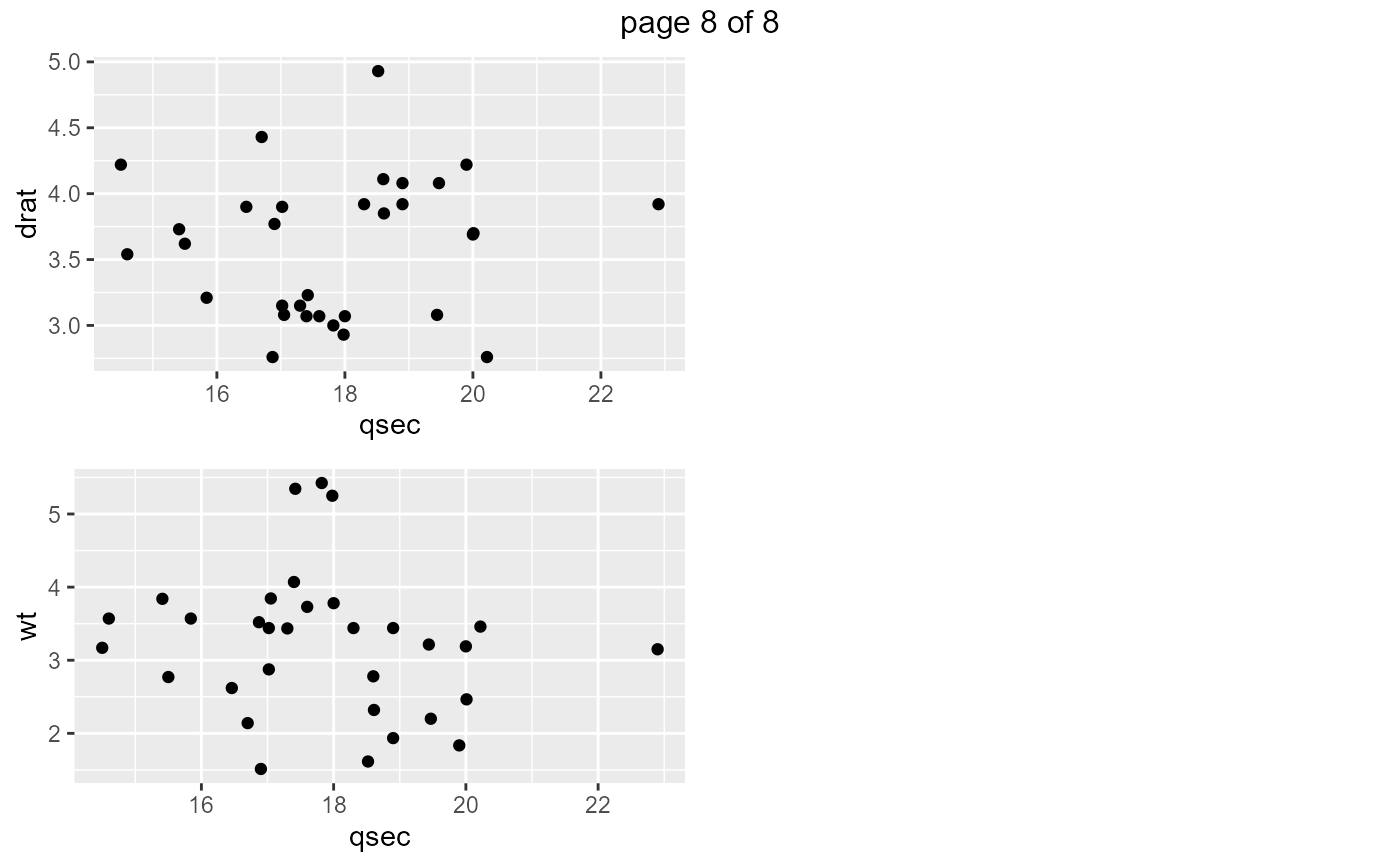Creates scatter plots if the data has continuous variables.

ds_plot_scatter(data, ..., print_plot = TRUE)

## Arguments

data A data.frame or tibble. Column(s) in data. logical; if TRUE, prints the plot else returns a plot object.

## Examples

# plot select variables
ds_plot_scatter(mtcarz, mpg, disp)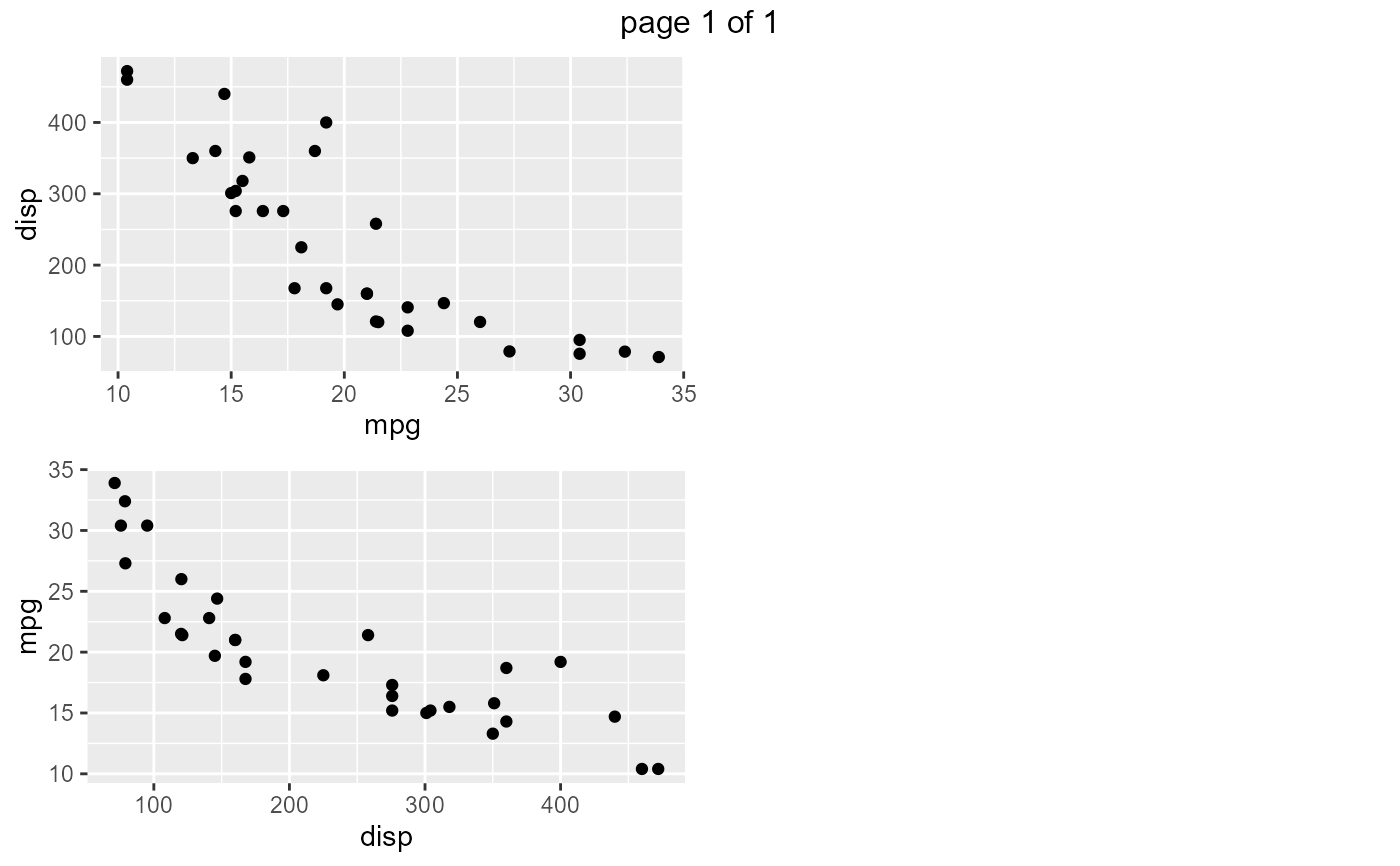# plot all variables
ds_plot_scatter(mtcarz)